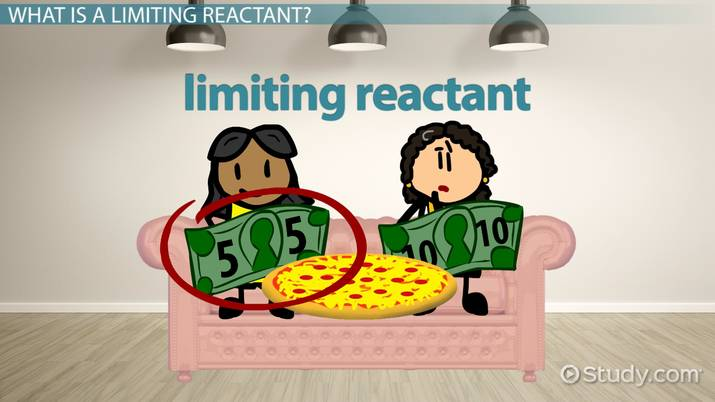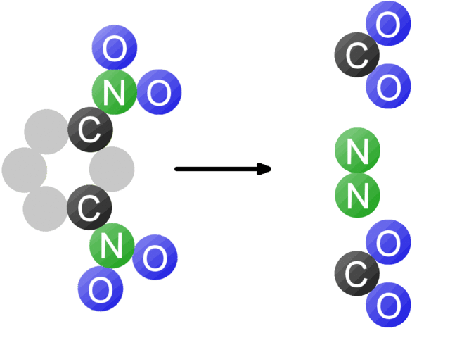# What is a Limiting Reactant?: Formula and Examples

Joseph Comunale, Laura Foist
• Author
Joseph Comunale

Joseph Comunale obtained a Bachelor's in Philosophy from UCF before becoming a high school science teacher for five years. He has taught Earth-Space Science and Integrated Science at a Title 1 School in Florida and has Professional Teacher's Certification for Earth-Space Science.

• Instructor
Laura Foist

Laura has a Masters of Science in Food Science and Human Nutrition and has taught college Science.

Learn the definition of a limiting reactant, the formula, and how to determine a limiting reactant. See examples of limiting reactants and their problems. Updated: 10/15/2021

Show

## Limiting Reactant Definition

Within chemistry and stoichiometry, there are limiting factors or variables that determine outcomes known as limiting reactants. Limiting reactants are ingredients within a chemical reaction that limit the amount of product made during a chemical reaction. Within a chemical reaction, the element or compound that is exhausted or consumed first is the limiting reactant. Limiting reactants are also sometimes called limiting reagents.

Chemical reactions require certain ratios of substances that act as fuel that maintain the reaction. If one of those substances is exhausted or consumed entirely, the chemical reaction cannot be sustained and therefore ceases, only yielding a certain amount of a product, while an excess reactant remains. An excess reactant is the reactant left over after the reaction stops when the limiting reactant is completely consumed. Within chemical reactions there is always a limiting reactant and an excess reactant unless the ratios of the reactants are perfectly balanced. The only way to determine which of the substances is the limiting reactant and which is the excess reactant is to compare their ratios to a balanced chemical equation that represents the chemical reaction those substances are about to undergo.An error occurred trying to load this video.

Try refreshing the page, or contact customer support.

Coming up next: Limiting Reactant Practice Problems

### You're on a roll. Keep up the good work!

Replay
Your next lesson will play in 10 seconds
• 0:03 What Is a Limiting Reactant?
• 0:58 Calculating the…
• 4:21 Lesson Summary
Save Save

Want to watch this again later?

Timeline
Autoplay
Autoplay
Speed Speed## Determining Limiting Reactant

It is important to determine the limiting reactant in a chemical reaction because it also determines how much of the product will result from the reaction, and how much of the excess reactant will be left over. There are two ways of determining which reactant is the limiting reactant: by comparing the ratio of reactants to each other next to a balanced chemical equation or by comparing the product amounts that can be produced by each reactant.

### Using the Comparison of Reactant

The comparison of reactant ratios to a balanced chemical equation is the most useful method when trying to determine the limiting reactant when there are only two reactants. To do this, the amounts of each reactant given in grams must be compared to the mole ratio of the reactants in the balanced version of the chemical reaction. For example, consider the following balanced chemical equation that represents the chemical reaction between propane ({eq}C_3H_8 {/eq}) and oxygen ({eq}O_2 {/eq}) which yields water ({eq}H_2O {/eq}) and carbon dioxide ({eq}CO_2 {/eq}).

{eq}C_3H_8 + 5O_2 → 4H_2O +3CO_2 {/eq}

Looking at the chemical reaction above, the coefficients in front of each molecule represent how many units or moles of that molecule are present before and after the chemical reaction. The equation expresses that 1 mole of propane combined with 5 moles of oxygen yields 4 moles of water and 3 moles carbon dioxide. Consider that a chemist studying this reaction will be provided with propane and oxygen. This means that for every 1 mole of propane, 5 moles of oxygen are needed to produce 3 moles of carbon dioxide. So, the ratio of propane to oxygen needs to be 1:5 to be balanced and not have any excess reactant. However, one mole of propane does not weigh the same as one mole of oxygen. So, it becomes difficult to use this ratio when working with actual substances.

Consider a chemist is provided propane and oxygen and needs to determine the limiting reactant. The chemist can't count the moles within each sample, but the chemist can weigh each of the substances. Suppose the chemist is given 10 grams of propane and 15 grams of oxygen. The chemist should follow the following steps to determine which substance will be the limiting reactant:

Step 1: First determine the molecular weights of each mole because a mole of propane doesn't weigh the same as a mole of oxygen. Molecular weight is how many grams (or amu) of an element or compound is found in a mole of that element or compound.

Propane is made up of 3 carbon atoms and 8 hydrogen atoms. The atomic mass of a carbon atom is 12 atomic mass units, and the atomic mass of a hydrogen atom is 1 atomic mass unit. Therefore, for every molecule of propane, there are 44 atomic mass units.

A molecule of oxygen is made up of 2 oxygen atoms. The atomic mass of oxygen is 16 amu, therefore one oxygen molecule is equal to 32 amu. The chemical reaction requires 5 moles of oxygen and therefore requires 160 amu of oxygen.

Step 2: Determine the ratio of atomic mass units between each of the reactants in the balanced chemical equation.

Therefore, for every 44 amu of propane, there needs to be 160 amu of oxygen for the reaction to be sustained. Therefore, the ratio needs to be 3.63:1 or 3.63 amu of oxygen for every 1 amu of propane.

Step 3: Compare the ratio of atomic mass units to the ratio the chemist is provided in grams.

The ratio of 3.63:1 amu can be scaled up to grams and still work. So, if the chemist is provided 15 grams of oxygen and 10 grams of propane, the ratio is 1.5:1 in grams. Since the oxygen ratio is 1.5 instead of the needed 3.63, there is not enough oxygen to sustain the chemical reaction long enough to consume all the propane. Therefore, oxygen is the limiting reactant, and propane is the excess reactant.

### Using the Comparison of Product

Another strategy that also works involves the chemist calculating how much product each reactant will produce. The reactant that produces the least amount of product is the limiting reactant. To do this, the chemist will still have to consider the molecular weights of the reactants. For every mole of propane, the chemical reaction yields 3 moles of carbon dioxide. And for every 5 moles of oxygen, the chemical reaction also yields 3 moles of carbon dioxide.

Carbon dioxide is made up of 1 carbon atom and 2 oxygen atoms. Carbon has an atomic mass of 12 amu, and oxygen has an atomic mass of 16 amu. Therefore, 1 mole of carbon dioxide is equal to 44 amu. The balanced chemical equation has 3 moles of carbon dioxide and therefore 132 amu of carbon dioxide. Considering the math from the previous example, this means that for every 44 amu of propane, and 160 amu of oxygen, 132 amu of carbon dioxide is produced in the chemical reaction. Again, this ratio would be the same in grams since atomic mass units and grams are both units for mass. Therefore, amu can simply be represented as grams.

For this example, the chemist is provided 25 grams of propane and 150 grams of oxygen. In this example, grams need to be converted into moles, and therefore amu per mole can be represented as grams per mole. Consider the balanced equation again:

{eq}C_3H_8 + 5O_2 → 4H_2O +3CO_2 {/eq}

Step 1: Convert the grams of the provided ingredients into moles.

Converting 25 grams of propane to moles:

{eq}Mol C_3H_8 = \frac{grams C_3H_8}{g/mol C_3H_8} = \frac{25 g}{44g/mol} = 0.5681 mol {/eq}

Converting 150 grams of oxygen to moles:

{eq}Mol O_2 = \frac{grams O_2}{g/mol O_2} = \frac{150 g}{32 g/mol} = 4.6875 mol {/eq}

Step 2: Multiply the moles of each reactant to their corresponding ratio with the product carbon dioxide. This will determine the amount of moles of carbon dioxide each reactant will produce.

Amount of moles of carbon dioxide produced by 25 grams of propane:

{eq}Mol CO_2 = 0.5681 mol C_3H_8 \cdot \frac{3 mol CO_2}{1 mol C_3H_8} = 1.7043 mol CO_2 {/eq}

Amount of moles of carbon dioxide produced by 150 grams of oxygen:

{eq}Mol CO_2 = 4.6875 mol O_2 \cdot \frac{3 mol CO_2}{5 mol O_2} = 2.8125 mol CO_2 {/eq}

To unlock this lesson you must be a Study.com Member.

#### How do you define a limiting reactant?

A limiting reactant is defined as an ingredient or reactant in a chemical reaction that produces the least amount of the product. In other words, the limiting reactant is the reactant that is exhausted or consumed entirely first, ceasing the chemical reaction. The chemical reaction can only be sustained for as long as its fuel or reactants are present.

#### What is a limiting reactant and how is it determined?

The limiting reactant is a reactant or ingredient in a chemical reaction that is exhausted or consumed first for the length the chemical reaction is sustained. The limiting reactant can be determined if the molecular weight of the reactants and products are known, and if the amounts of the reactants are compared to a balanced chemical equation.

### Register to view this lesson

Are you a student or a teacher?